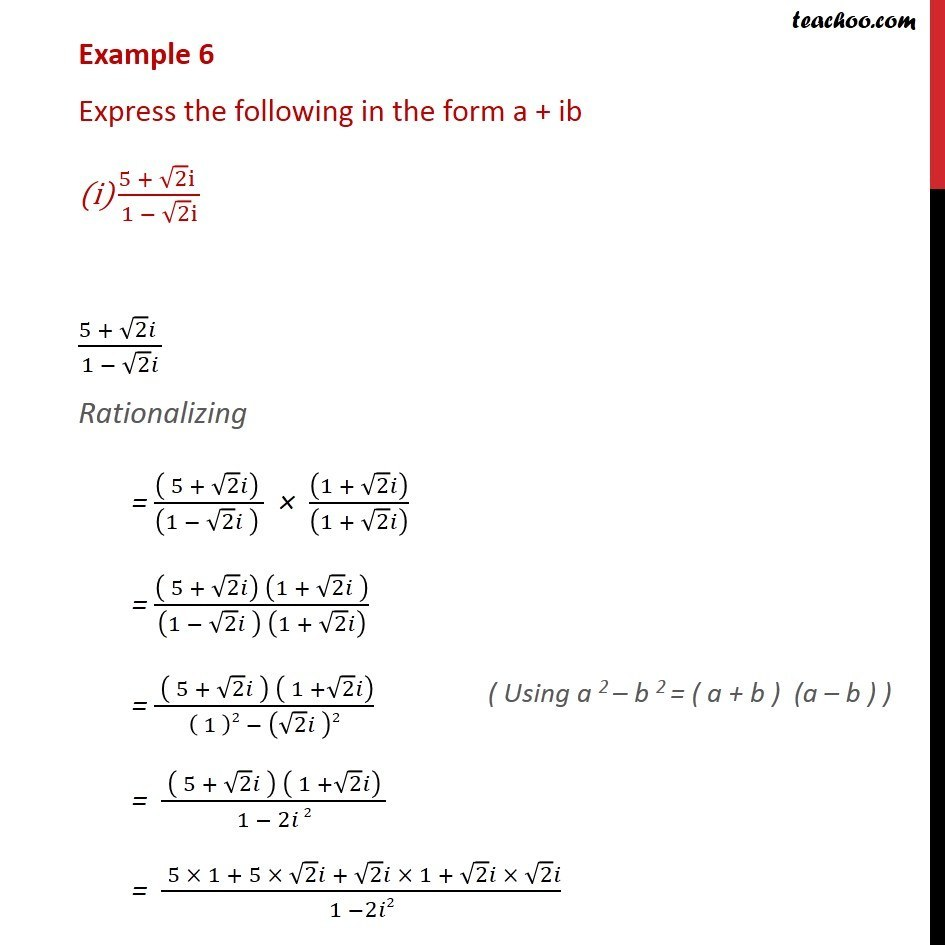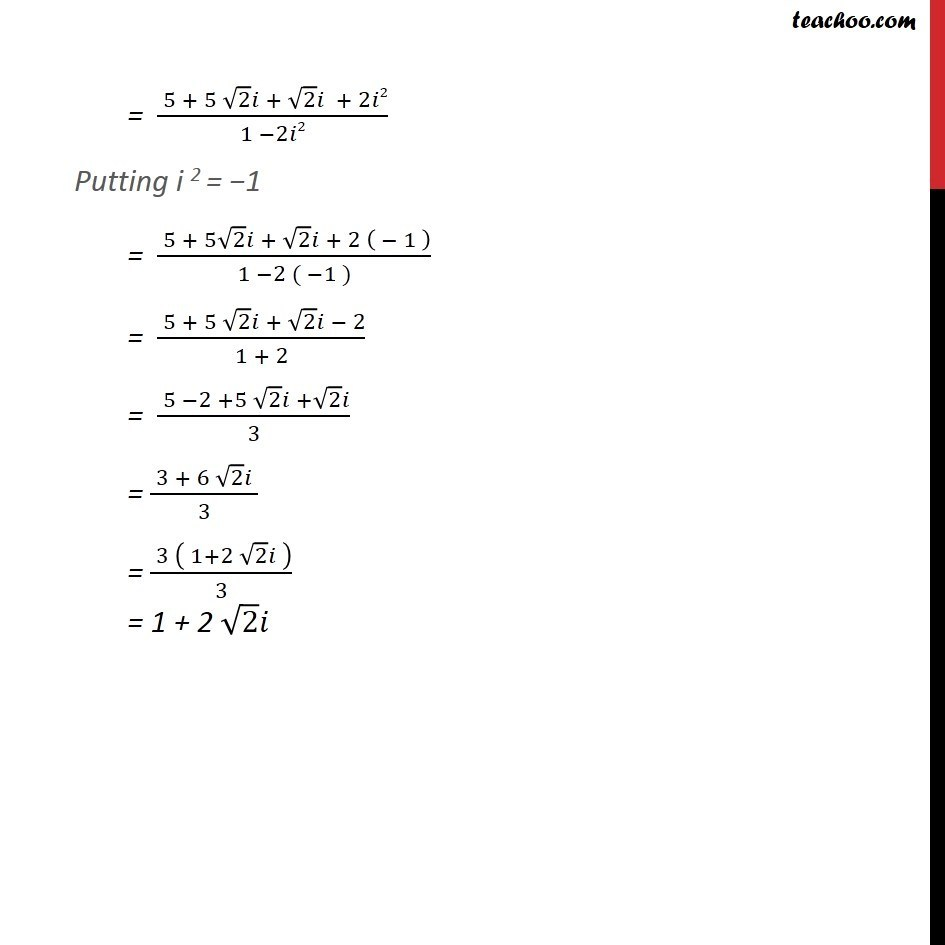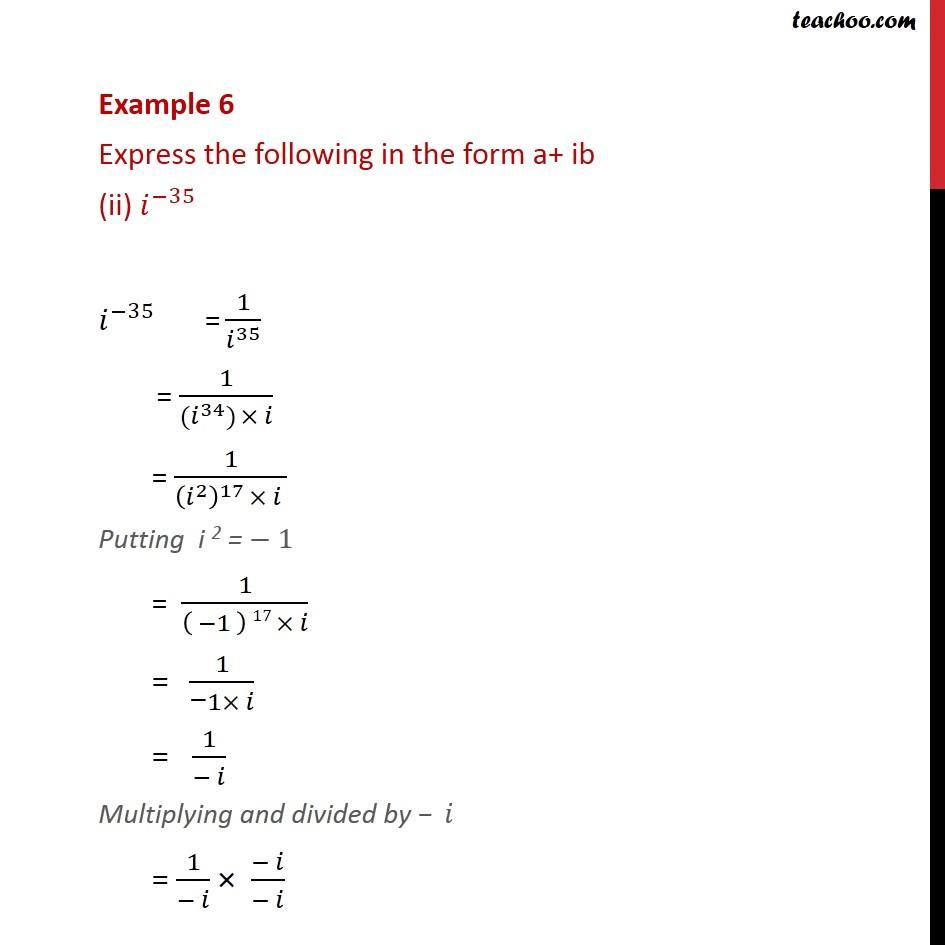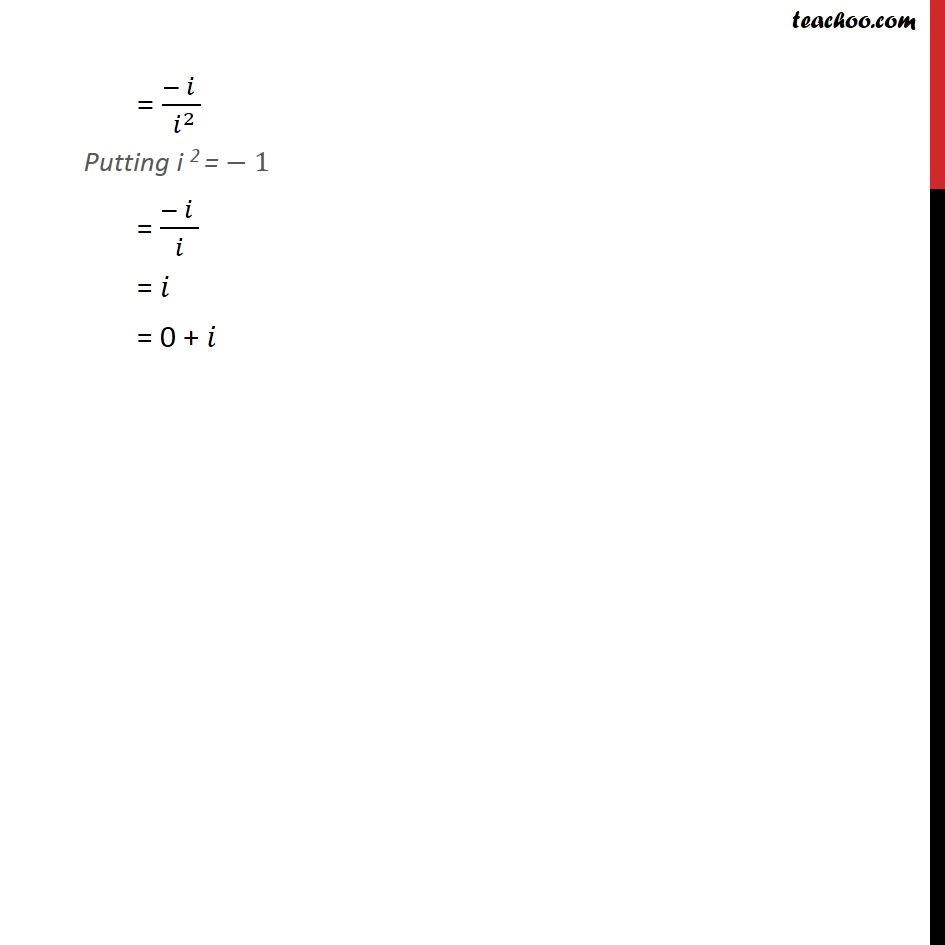Subscribe to our Youtube Channel - https://you.tube/teachoo

1. Chapter 5 Class 11 Complex Numbers
2. Serial order wise
3. Examples

Transcript

Example 6 Express the following in the form a + ib (5 + 2 i )/(1 2 i) (5 + 2 )/(1 2 ) Rationalizing = (( 5 + 2 ) )/((1 2 ) ) ((1 + 2 ))/((1 + 2 ) ) = (( 5 + 2 ) (1 + 2 ))/((1 2 ) (1 + 2 ) ) = ( ( 5 + 2 ) ( 1 + 2 ))/(( 1 )2 ( 2 )2) = ( ( 5 + 2 ) ( 1 + 2 ) )/(1 2 2) = ( 5 1 + 5 2 + 2 1 + 2 2 )/(1 2 2) = ( 5 + 5 2 + 2 + 2 2)/(1 2 2) Putting i 2 = 1 = ( 5 + 5 2 + 2 + 2 ( 1 ))/(1 2 ( 1 )) = ( 5 + 5 2 + 2 2)/(1 + 2) = ( 5 2 +5 2 + 2 )/3 = ( 3 + 6 2 )/3 = ( 3 ( 1+2 2 ))/3 = 1 + 2 2 Example 6 Express the following in the form a+ ib (ii) ^( 35) ^( 35) = 1/ ^35 = 1/( ( ^34) ) = 1/(( ^2 )^17 ) Putting i 2 = 1 = 1/(( 1 ) 17 ) = 1/(" " 1 ) = 1/( ) Multiplying and divided by = 1/( ) ( )/( ) = ( )/( ^2 ) Putting i 2 = 1 = ( )/( ) = = 0 +

Examples